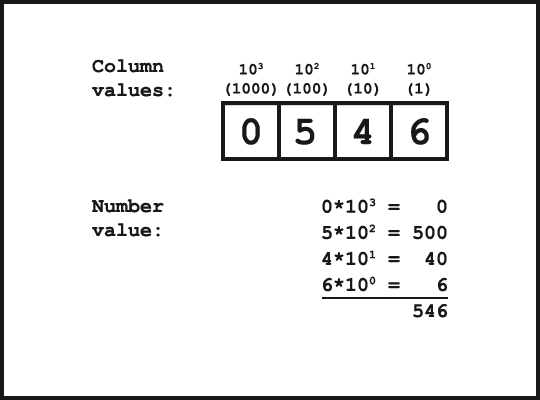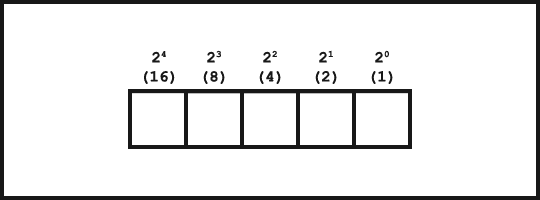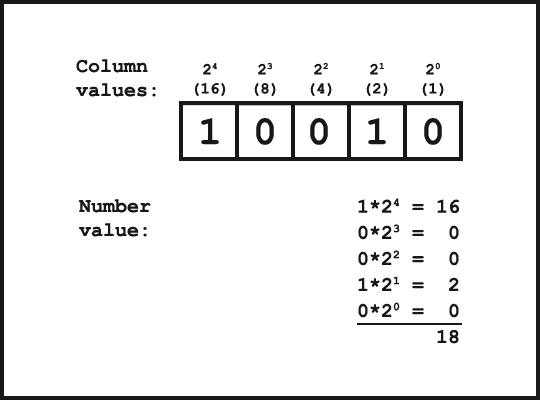Jack Morris
An Introduction to Binary Numbers
29 Sep 2014

In our standard base-10 counting system, we use the digits 0 through 9 to write numbers. This makes sense and seems natural to us, as it's what we're used to and it's what we were taught in school. However, base-10 isn't some magical system that the universe is built on, and there's no special rule that states that it's the counting system we have to use. It makes just as much sense to count things in different bases such as base-8 or even base-16 (using 16 different characters!) if it simplifies a certain application, or even if you just feel like it.

One alternative counting system however has seen a major rise to prominence over the last half century, and that's the binary numeral system, or base-2. Base-2 uses two characters to represent numbers: 0 and 1. This makes it an easy way to represent numbers in devices consisting of components that can exhibit one of two states, letting one state represent a 0, and the other a 1. One such device is the one that you're currently reading this post on - unless you like it so much that you've printed it out that is.

Practically all modern electronic devices represent numbers internally using base-2, explaining the massive rise in interest in binary. It's also a good reason why anyone interested in electronics, programming, or hardware design in general should at least have a basic understanding of how to make sense of it. These basics aren't too hard to get your head around.

Let's start with something familiar: reading base-10 numbers. When counting in base-10 each digit in the number has a value that's dictated by its location within the number. By seperating the number into 'columns', you have the ones column, the tens column, hundreds column, and so on. As you may have noticed, the values of each column are increasing powers of 10, that it `10^0` (1), `10^1` (10), and `10^2` (100). To determine the value of a number in base-10, you multiply each digit by the value of its column, and add up the results.I'm assuming that this isn't news to you, so why am I going on about it? Well, the process for converting binary numbers into base-10 so you can read them is completely similar, aside from the value of each column. Where as with base-10 each column is worth an increasing power of 10 (first column: `10^0`, second column: `10^1`, ...), in base-2, each column is worth an increasing power of 2.Hence, calculating the value of a binary number involves taking the digit in each column of the number (either a 0 or a 1), multiplying it by the value of the column (a power of 2), then adding all of these results together. By remembering the powers of 2 involved, this can be done reasonably easily in your head since each multiplication is only by 0 or 1.

As an example, take the binary number 10010. As shown below, this has the value of 18 when converted into base-10.# Going the Other Way

Converting a base-10 number into its binary representation has a different method. The method I use to do this in my head is easy once you remember some powers of 2: looking at the base-10 number, going downwards in powers of 2 from the largest one that's smaller than it, ask 'is this power of 2 smaller than my number?'. If so, stick a 1 in the column corresponding to that power, and if not, a 0. Then subtract the power of two from your number, and continue. By the time you get to the last column, the number you're converting will be down to 0, and your binary number will be complete.

Let's go through an example, converting 13 into binary:

``````Initial number: 13
Largest power of 2 under 13:

3rd column:
column value = 2^3 = 8
8 is less than 13:
1st column = 1
new number = 13 - 8 = 5

2nd column:
column value = 2^2 = 4
4 is less than 5:
2nd column = 1
new number = 5 - 4 = 1

1st column:
column value = 2^1 = 2
2 isn't less than 1:
3rd column = 0
number isn't changed

'0th' column:
value = 2^0 = 1
1 is equal to 1:
4th column = 1
new number = 1 - 1 = 0
(we're done!)

Hence, 13 in binary = 1101
``````

You can see how this is essentially the opposite of what we were doing before. As we can have at most one of each column (each column value is either multiplied by 0 or 1), we go through in decreasing value of columns and see which ones are needed.

# Wrap Up

Both of these techniques work when converting any number base to/from base-10, not just base-2. However binary is an interesting example as it pops up so often when dealing with the inner workings of computer hardware.